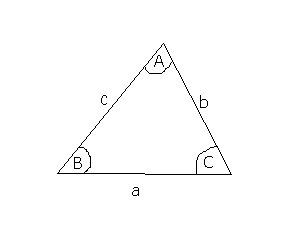## Sine and Cosine Rule

The Sine Rule

The sine rule is an important rule relating the sides and angles of any triangle (it doesn't have to be right-angled!):

If a, b and c are the lengths of the sides opposite the angles A, B and C in a triangle, then:a = b = c sinA sinB sinC

If you wanted to find an angle, you can write this as:

 sinA = sinB = sinC a b c

The Cosine Rule

This also works in any triangle:

c2 = a2 + b2 - 2abcosC
which can also be written as:
a2 = b2+ c2 - 2bccosA

The area of a triangle

The area of any triangle is ½absinC (using the above notation).
This formula is useful if you don't know the height of a triangle (since you need to know the height for ½ base × height).